# Introduction To Equations Of Circles

Go back to  'Circles'

As we know very well from pure geometry, a circle is a geometrical figure described by a moving point in the Euclidean plane such that its distance from a fixed point is always constant. The fixed point is called the center of the circle while the fixed distance is called the radius of the circle.

Moving over to a co-ordinate system, let us denote the center $$C$$ of the circle by $$({x_0}, {y_0})$$ and the radius by $$r$$. For any point $$P(x , y)$$ lying on the circle, the length $$PC$$ must be equal to $$r$$. Using the distance formula for $$PC ,$$ we therefore obtain:

$\boxed{{{\left( {x - {x_0}} \right)}^2} + {{\left( {y - {y_0}} \right)}^2} = {r^2}}:{\text{Equation}}\,{\text{of}}\,{\text{the}}\,{\text{circle}}$

This equation must be satisfied by every point$$P(x , y)$$ lying on the circle; therefore, this is the equation that uniquely describes the given circle. We simply call it the equation of the circle, with center $$(x_0 , y_0 )$$ and   radius $$r$$.

For example, consider the circle with its center at $$(1, 1)$$ and radius equal to unity: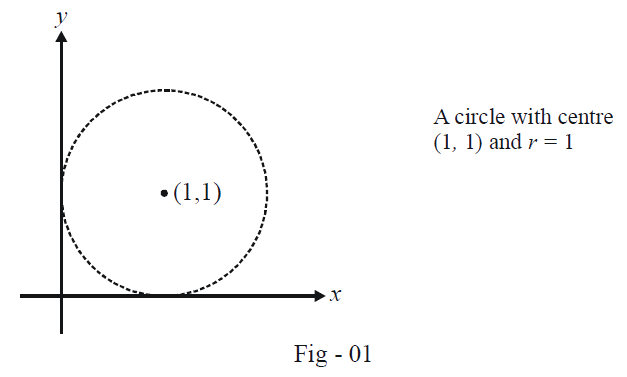The equation of the this circle is

\begin{align} {\left( {x - 1} \right)^2} + {\left( {y - 1} \right)^2} &= {1^2} \hfill \\ \Rightarrow \qquad {x^2} + {y^2} - 2x - 2y + 1 &= 0 \hfill \\ \end{align}

Example - 1

Find the equation of the circle passing through the points $$(0, 0), (3, 0)$$and $$(1, 2)$$.

Solution: To write the equation of the required circle, we must find its center and radius.

Recall from pure geometry that a circle can always be drawn through three non-collinear points. This can be done as follows: join the points to form a triangle. Draw the perpendicular bisectors of any of the two sides of this triangle. Their point of intersection gives us the center$$C$$. The distance of $$C$$ from any of the vertices gives the radius $$r$$ of the circles: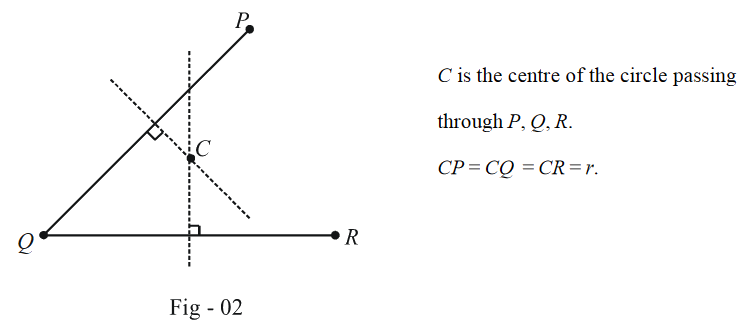We apply this result to the current example: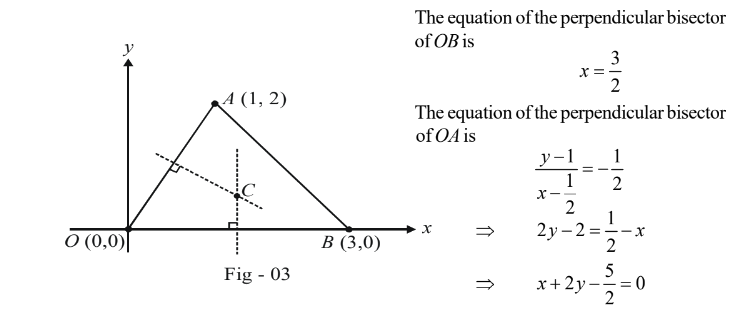The point $$C$$ is the intersection of the two angle bisectors

\begin{align}\Rightarrow \qquad & C \equiv \left( {\frac{3}{2},\frac{1}{2}} \right)\end{align}

We can now easily evaluate the radius r as the length $$OC$$:

\begin{align}r = OC = \sqrt {\frac{9}{4} + \frac{1}{4}} = \frac{{\sqrt {10} }}{2}\end{align}

Finally, the equation of the required circle becomes:

\begin{align}{\left( {x - \frac{3}{2}} \right)^2} + {\left( {y - \frac{1}{2}} \right)^2} = \frac{{10}}{4}\end{align}

We will subsequently see another method to solve this type of questions.

Example - 2

Find the equation of the circle which touches the co-ordinate axes and whose centre lies on the \begin{align}x - 2y = 3\end{align} line

Solution: A circle of radius r touching the co-ordinate axes can be in one of the four following configurations, with four corresponding equations mentioned alongside: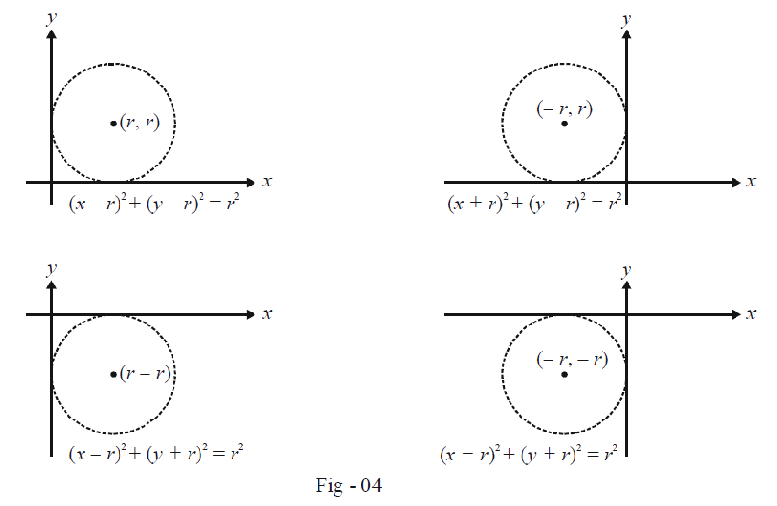Note from these four possible cases that the centre of such a circle either lies on $$y = x$$or on $$y = - x$$.

In the current example, the centre is also given to lie on $$x – 2y = 3$$. Thus, there will be two circles, with the two centres being given by the point of intersection $$y = x$$ and $$y = - x$$ with $$x – 2y = 3.$$

\begin{align} y = x\,{\rm{and}}\,\,x - 2y = 3 & \Rightarrow \qquad {C_1} \equiv \left( { - 3, - 3} \right)\\ &\Rightarrow \qquad {\rm{Equation}}\;{\rm{of}}\;{\rm{the}}\;{\rm{circle}}\;{\rm{is}}\;{\left( {x + 3} \right)^2} + {\left( {y - 3} \right)^2} = 9\\ y = - x\,{\rm{and}}\,\,x - 2y = 3 & \Rightarrow \qquad {C_2} \equiv \left( {1,\, - 1} \right)\\ & \Rightarrow \qquad {\rm{Equation}}\;{\rm{of}}\;{\rm{the}}\;{\rm{circle}}\;{\rm{is}}\;{\left( {x - 1} \right)^2} + {\left( {y + 1} \right)^2} = 1 \end{align}

Example - 3

Find the equation of the circle with radius 5 and which touches another circle \begin{align}{x^2} + {y^2} - 2x - 4y - 20 = 0\end{align} externally at the point $$(5, 5)$$

Solution: Let us first try to rearrange the equation of the given circle in the standard form from which we’ll be able to deduce its centre and radius:

\begin{align} & {x^2} + {y^2} - 2x - 4y - 20 = 0\\ \Rightarrow \qquad & {\left( {x - 1} \right)^2} + {\left( {y - 2} \right)^2} = 25 \end{align}

Therefore, the centre of this circle is $$(1, 2)$$ and its radius is $$5$$.

We should now draw a geometrical figure which will certainly make things more clear: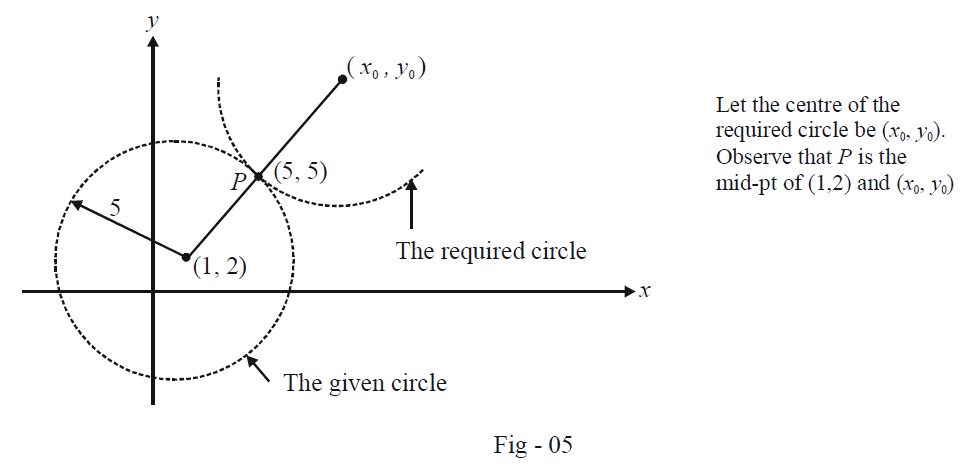As explained in the figure, we can now evaluate \begin{align}({x_0},\;{y_0})\end{align}, the centre of the required circle:

\begin{align}\frac{{{x_0} + 1}}{2} = 5\,\, \Rightarrow \,\,{x_0} = 9 \qquad \qquad \qquad \frac{{{y_0} + 2}}{2} = 5\,\, \Rightarrow \,\,{y_0} = 8\end{align}

Thus, the required equation is

${\left( {x - 9} \right)^2} + {\left( {y - 8} \right)^2} = 25$